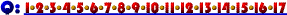4. Suppose the Levee Blue Jeans® company uses excessive quantities of Cheap Blue Dye® in the production of its new, hip line of Irritatingly Stiff Jeans®. If the wholesale cost of Cheap Blue Dye® increases, which cost curves will shift upward for Irritatingly Stiff Jeans®.

1. Average fixed cost.
2. Marginal cost and average total cost.
3. Average total cost, average fixed cost, average variable cost and marginal cost.
4. Average total cost, average variable cost and marginal cost.
5. Average total cost and average variable cost.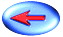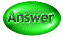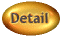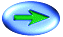Click Here if you don't see topics listed to the left and below.